Win up to 100% scholarship on Aakash BYJU'S JEE/NEET courses with ABNAT Win up to 100% scholarship on Aakash BYJU'S JEE/NEET courses with ABNAT

# JEE Main 2021 July 20 Shift 2 Chemistry Question Paper

JEE Main 2021 July 20 shift 2 Chemistry solved paper is a perfect study resource for the candidates who are appearing for the entrance exam. By going through the question paper and solving the questions they will get a better idea of the paper pattern, question types and the difficulty level of the exam.

### JEE Main 2021 July 20 Shift 2 Chemistry Question Paper

More significantly, our subject experts have examined the JEE Main 2021 Chemistry Paper of July 20 shift 2 and prepared solutions accordingly. The solutions are given in a detailed manner and in a step by step format. This will help candidates to gain a crystal clear understanding of the topics as well as develop the right approach to solving the different problems. The Chemistry question paper uploaded at BYJU’S will also enable the students to obtain a fair idea about the distribution of marks with respect to each topic. All in all, going through and practising these questions will help them know the amount of preparation that will be required to face the examination.

July 20 Shift 2 – Chemistry Question Paper and Solutions

Question 1. Which of the following gases retards the rate of photosynthesis?

a. CO
b. NO2
c. CO2
d. CFC

When fossil fuel is burnt nitrogen and dioxygen combine to yield significant quantities of nitric oxide (NO) and nitrogen dioxide (NO2) as given below:

N2 (g) + O2 (g) → 2NO (g)

2NO (g) + O2 (g) → 2NO2 (g)

Higher concentrations of NO2 damage the leaves of plants and retard the rate of photosynthesis.

Hence, option (b) is the correct answer.

Question 2. The correct order of rate of hydrolysis of ester, acid chloride, and acid anhydride is:

a. Acid chloride > Acidic anhydride > Ester
b. Ester > Acidic anhydride > Acid chloride
c. Ester > Acid chloride > Acidic anhydride
d. Ester < Acid chloride < Acidic anhydride

Hydrolysis of ester, acid chloride and acid anhydride follows a nucleophilic addition mechanism wherein water acts as a nucleophile and it attacks the carbonyl carbon of the reactant. So, the reactant with higher δ+ charge on carbonyl carbon will be more readily attacked by water.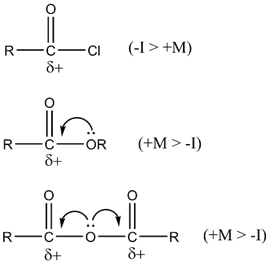Acid chloride > Acidic anhydride >Ester

Hence, option (a) is the correct answer.

Question 3. The difference in the number of unpaired electrons in NiCl2 and [Ni(CN)6]2-is:

a. 0
b. 1
c. 2
d. 3

In NiCl2, Ni is in +2 oxidation state.

Hence, its electronic configuration is 3d8.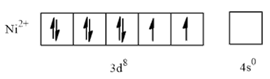Thus, the number of unpaired electrons in NiCl2 is 2.

In [Ni(CN)6]2-, Ni is in a +4 oxidation state and CN is a strong field ligand.

Hence, its electronic configuration is 3d6.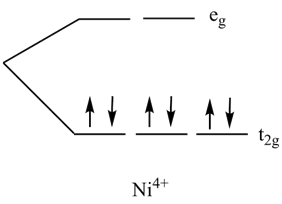Thus, the number of unpaired electrons in [Ni(CN)6]2- is 0.

Therefore, the difference in the number of unpaired electrons in NiCl2 and [Ni(CN)6]2- is 2.

Hence, option (c) is the correct answer.

Question 4. 4s2 4p1 Identify the element that is diagonally a part of the next period in p-block.

a. Ge
b. Sn
c. In
d. Pb

The element with the outer electron configuration of 4s2 4p1 is Gallium (Ga). Therefore, diagonally the next period in the p-block will have an outer electron configuration of 5s2 5p2.

The element corresponding to this configuration is Tin (Sn).

Hence, option (b) is the correct answer.

Question 5. Find product B.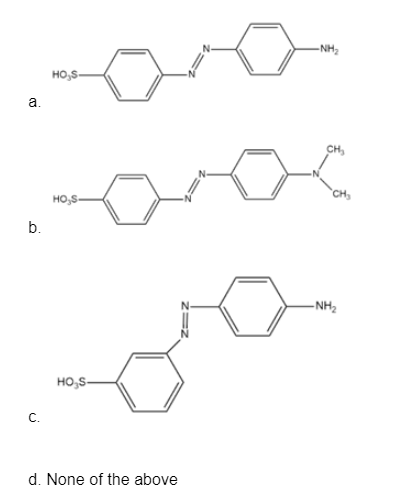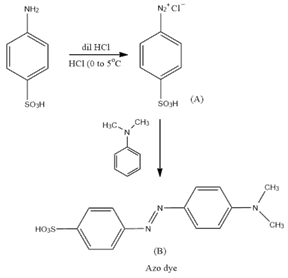Hence, option (b) is the correct answer.

Question 6. In FCC, 50% of tetrahedral voids are filled. Find the effective number of atoms in a unit cell if it consists of the same atoms in the lattice and voids.

a. 6
b. 8
c. 4
d. 5

In CCP/FCC, there are two tetrahedral voids on every body that is diagonal of the unit cell. FCC has a total of 4 body diagonals. Thus, a total of 8 tetrahedral voids are present inside the unit cell. Also, the effective number of atoms in the FCC arrangement is 4.

Therefore, the effective number of atoms in the new unit cell is equal to the sum of the effective number of atoms in FCC and the number of atoms in tetrahedral voids.

Zeff = 4 + 4

Zeff = 8

Hence, option (b) is the correct answer.

Question 7. Which of the following does not have a magnetic moment of 1.73 B.M?

a.

$$\begin{array}{l}O_{2}^{+}\end{array}$$

b.
$$\begin{array}{l}O_{2}^{-}\end{array}$$

c. [Cu(NH3)4]Cl2
d. CuI

Magnetic moment = √n(n+2) B.M

Where n indicates the number of unpaired electrons.

Species having one unpaired electron will have a magnetic moment of 1.73 B.M

Electronic configuration of O2

$$\begin{array}{l}O_{2} = KK(\sigma _{2S})^{2} (\sigma_{2S}^{\ast})^{2} (\sigma _{2P_{z}})^{2} (\pi_{2P_{x}})^{2} = (\pi_{2P_{y}})^{2} (\pi _{2P_{x}}^{\ast })^{1} = (\pi _{2P_{y}}^{\ast })^{1}\end{array}$$
$$\begin{array}{l}O_{2}^{+} = KK(\sigma _{2S})^{2} (\sigma_{2S}^{\ast})^{2} (\sigma _{2P_{z}})^{2} (\pi_{2P_{x}})^{2} = (\pi_{2P_{y}})^{2} (\pi _{2P_{x}}^{\ast })^{1} = (\pi _{2P_{y}}^{\ast })^{0}\end{array}$$
$$\begin{array}{l}O_{2}^{-} = KK(\sigma _{2S})^{2} (\sigma_{2S}^{\ast})^{2} (\sigma _{2P_{z}})^{2} (\pi_{2P_{x}})^{2} = (\pi_{2P_{y}})^{2} (\pi _{2P_{x}}^{\ast })^{2} = (\pi _{2P_{y}}^{\ast })^{1}\end{array}$$

The number of unpaired electrons in O_2^- is 1

In [Cu(NH3)4]Cl2, Cu is in a +2 oxidation state.

Outer electronic configuration of Cu is [Ar]3d104s1

Outer electronic configuration of Cu2+ is [Ar]3d94s0

Therefore, [Cu(NH3)4]Cl2 has one unpaired electron.

In CuI, Cu is in a +1 oxidation state.

Thus, the outer electronic configuration of Cu+ is [Ar]3d94s0

Therefore, CuI has no unpaired electron. So, it has zero magnetic moments.

Hence, option (d) is the correct answer.

Question 8. Benzene with Br and CONH2 at the meta position is made to react with KOBr to give A and the same reactant is made to react with LiAlH4 to give B. Predict products A and B.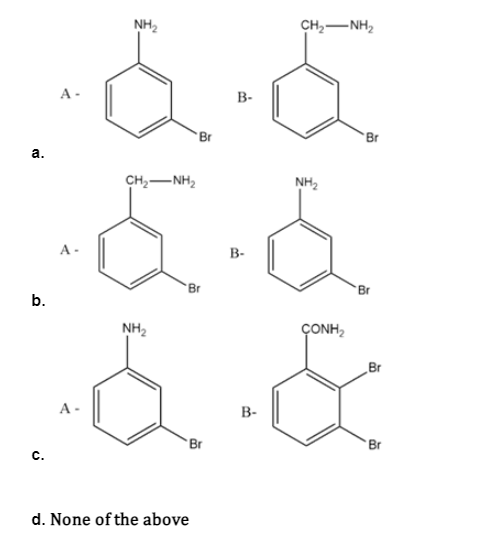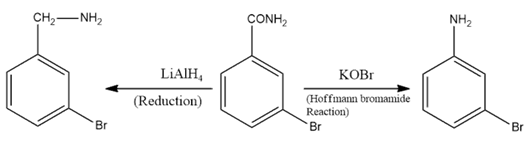Hence, option (a) is the correct answer.

Question 9. Predict the product.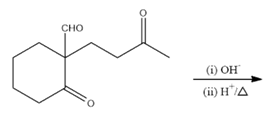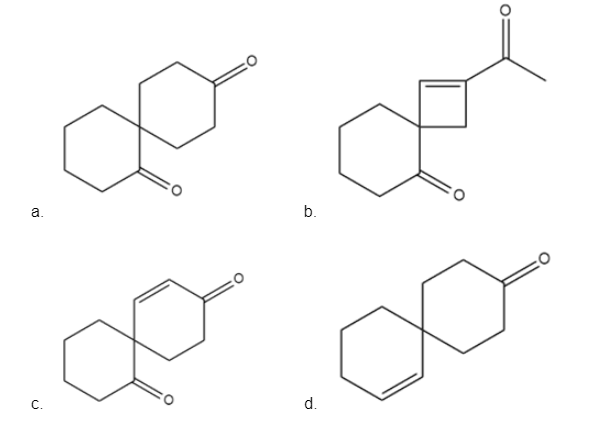This reaction is aldol condensation.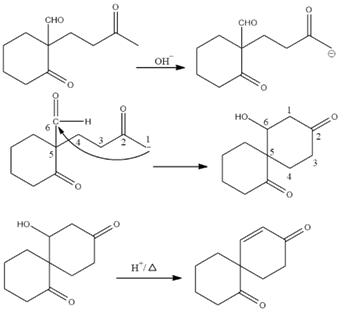Hence, option (c) is the correct answer.

Question 10. Sodium does not normally react with:

a. Ammonia gas
b. Ethyne
c. But-2-yne
d. Propyne

For reaction with sodium metal, the compound should have acidic hydrogen. Ethyne and propyne have terminal acidic hydrogen so they react with sodium metal. Ammonia gas undergoes a reaction with sodium to form sodamide with the release of hydrogen gas.

But-2-yne lacks terminal acidic hydrogen so it does not react with Na.

Hence, option (c) is the correct answer.

Question 11. The hybridization of N in

$$\begin{array}{l}NO_{2}^{-}\end{array}$$
,
$$\begin{array}{l}NO_{2}^{+}\end{array}$$
,
$$\begin{array}{l}NO_{4}^{+}\end{array}$$
are respectively.

a. sp2, sp, sp3
b. sp3, sp, sp3
c. sp, sp, sp3
d. sp2, sp2, sp3

To find the number of hybrid orbitals involved in hybridization, the formula is as follows:

H = ½ [V + M – C + A]

Where,

H is the number of orbitals involved in hybridization

V is the number of valence electrons on the central atom

M is the number of monovalent atoms attached to the central atom

C is the charge on the cation

A is the charge on the anion

For

$$\begin{array}{l}NO_{2}^{-}\end{array}$$

H = (5+1) / 2 = 3

sp2 hybridization

For

$$\begin{array}{l}NO_{2}^{+}\end{array}$$

H = (5 – 1) / 2 = 2

sp hybridization

For

$$\begin{array}{l}NO_{4}^{+}\end{array}$$

H = (5 + 4 – 1) / 2 = 4

sp3 hybridization

Hence option (a) is the correct answer.

Question 12. In nitration HNO3 and H2SO4 act as:

a. Both acid
b. Both base
c. HNO3: acid and H2SO4: base
d. HNO3: base and H2SO4: acid

Nitration using HNO3 and H2SO4 goes by an electrophilic substitution mechanism.

In this process H2SO4 acts as an acid whereas HNO3 acts as base as shown: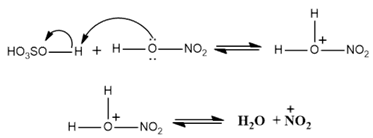Hence option (d) is the correct answer.

Question 13. Bakelite is formed by cross-linking of which of the following:

a. Novolac
b. Buna-S
c. Dacron
d. PHBV

Bakelite is formed by cross-linking of novolac and formaldehyde.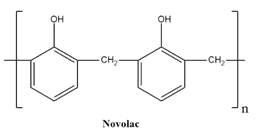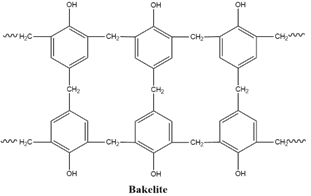Hence option (a) is the correct answer.

Question 14. The initial mass of a radioactive substance becomes 1/16th in 80 minutes. Find the half-life.

[A]t = [A]o / 2n

[A]t is the concentration at time t

[A]o is the initial concentration

n is the number of half-lives

Given:

$$\begin{array}{l}[A]_{t} = \frac{[A]_{o}}{16}. So, \frac{[A]_{o}}{2^{n}} = \frac{[A]_{o}}{16}\end{array}$$

1 / 2n = 1 / 16

n = 4

For 4 half-lives, time taken is 80 min.

For 1 half-life, time taken is 80/4 min.= 20 min.

Hence half-life is 20 min.

Question 15. An equimolar mixture of NaOH and Na2CO3 weighs 4 g, then the weight of NaOH is:

Let the weight of NaOH be w1 g and that of Na2CO3 be w2 g

The molar mass of NaOH is 40 g/mol

Molar mass of Na2CO3 is 106 g/mol

Given: w1 + w2 = 4 g

For an equimolar mixture:

$$\begin{array}{l}\frac{w_{1}}{40} = \frac{w_{2}}{106}\end{array}$$
, Solving for w1

w1 = 160/146 g = 1.096 g

Hence, the weight of NaOH is 1.096 g.

Question 16. The below given first-order reaction has initial and final moles of PCl5 as 50 and 10 respectively in 120 minutes. Find the rate constant. Given log10⁡ 5=0.693

PCl5 → PCl3 + Cl2

$$\begin{array}{l}k = \frac{2.303}{t} log_{10}\frac{[A]_{o}}{[A]_{t}}\end{array}$$
[A]t is the concentration of PCl_5 at time t

[A]t is the initial concentration of PCl_5

k is rate constant

$$\begin{array}{l}k = \frac{2.303}{t} log_{10}\frac{50}{10} = \frac{2.303\times 0.693}{120}\end{array}$$

K = 0.013 min-1

Question 17. Cu2+ salts react with KI and form:

a. Cu2I2
b. CuI
c. Cu(I3)2
d. CuI3

2Cu2+ + 4KI → Cu2I2 (s) + I2

But the complete reaction is:

2CuSO4 (aq) + 4KI(aq) → 2K2SO4 (aq) + Cu2I2 (s) + I2

Iodine, since present in its lowest oxidation state i.e., -1 acts as a reducing agent and reduces

Cu2+ to Cu+ . The white precipitate obtained is Cu2I2.

Hence, option (a) is the correct answer.

Question 18. What is added with HNO_3 in the Carius method?

a. H2SO4
b. AgNO3
c. HNO3
d. CaCO3

Carius method: A known mass of an organic compound is heated with fuming nitric acid in the presence of silver nitrate (AgNO3) contained in a hard glass tube known as a Carius tube.

Hence, option (b) is the correct answer.

Question 19. Identify the process in the given reactions

1. $$\begin{array}{l}ZnCO_{3} \overset{\Delta }{\rightarrow}ZnO + CO_{2}\end{array}$$
2. $$\begin{array}{l}ZnS + O_{2} \overset{\Delta }{\rightarrow}ZnO + SO_{2}\end{array}$$

a. A = roasting; B = calcination.
b. A = calcination; B = roasting.
c. A = B = roasting.
d. A = B = calcination.

Calcination: It is the process of converting ore into oxide ore in a limited supply of air. Calcination is usually carried out for carbonates, bicarbonates and hydroxides.

In reaction A, carbonate ore of Zn is heated in the absence of oxygen and thereby converting into its oxide ore (ZnO). So, this process is called calcination.

Roasting: It is the process of converting ore into its oxide ore by heating it in the presence of excess air. It is usually carried out for sulphide ores.

In reaction B, the sulphide ore of Zn in the presence of oxygen is converted into its oxide ore (ZnO). So, this process is called roasting.

Hence option (b) is the correct answer.

Question 20. Which among the following statements about enzymes is not correct?

a. Enzymes are non-specific
b. Enzymes are temperature and pH specific
c. Almost all enzymes are proteins
d. Enzymes act as a catalyst

Enzymes are biocatalysts. Almost all the enzymes are proteins. They are highly specific in their actions. They work best at specific conditions of temperature and pH.

Hence option (a) is the correct answer.

Question 21. In an octahedral complex of Fe2+ in a high spin state, what is the magnetic moment (spin only)?

a. 4.89 BM
b. 1.73 B
c. 0 BM<.p>
d. 3.87 BM

Electronic configuration of 26Fe2+ is [Ar]3d64s0

Since an octahedral complex in a high spin state is getting formed, the transition of electrons is preferred over pairing. Hence, the correct distribution of electrons is as shown below: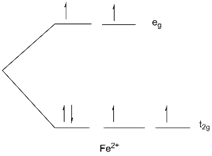Thus, the number of unpaired electrons (n) is 4.

Now the magnetic moment (µ)=√(n(n+2))

Putting the value of n

µ = √n(n+2) = √24 = 4.89 BM

Hence, the correct answer is option (a).

Question 22. Choose the correct structure of paracetamol.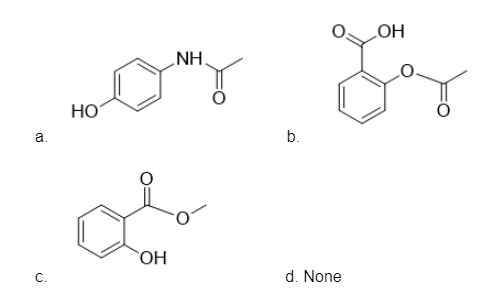The correct structure of paracetamol is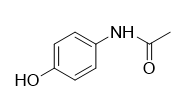Hence, the correct answer is option (a).

Question 23. What is the major use of dihydrogen (H2)?

a. Formation of HNO3
b. Synthesis of ammonia (NH3)
c. Fuel cell for generating electrical energy
d. Reduction of heavy metal oxides to metal

The major use of dihydrogen is in the synthesis of ammonia which then can be used in the manufacturing of nitric acid and nitrogenous fertilizers.

Hence, the correct answer is option (b).

Question 24. Product (P) is: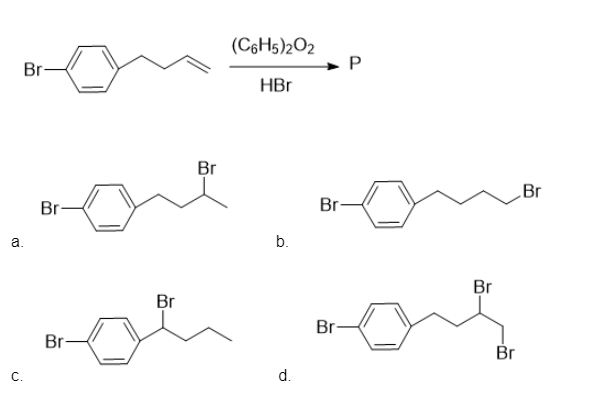The addition of HBr in the presence of peroxide takes place through the free radical addition mechanism. The product that is formed during the free radical addition mechanism is usually the anti-Markovnikov product. When HBr is added to unsymmetrical alkenes in the presence of peroxide, the Br is attached to that double-bonded carbon which contains more hydrogen atoms thereby forming an anti-Markovnikov addition product. This reaction is also known as the Kharash effect or peroxide effect.

Hence, the correct answer is option (b).

Question 25. Which of the following compounds are metamers?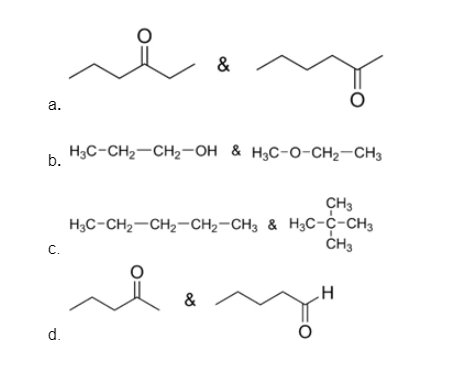Metamerism is a type of structural isomerism that is seen in a bivalent or polyvalent functional group. Metamers differ in the carbon chains that are linked to the bivalent or polyvalent functional groups.

Example: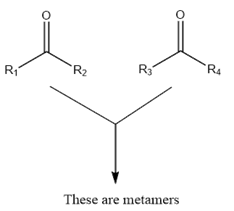Similarly in option (a),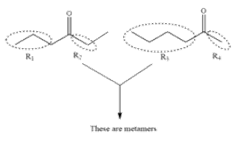Therefore, compounds given in option (a) are metamers. The compounds given in option (b) and (d) have different functional groups, therefore they are functional isomers, not metamers. The compounds given in option (c) are chain isomers.